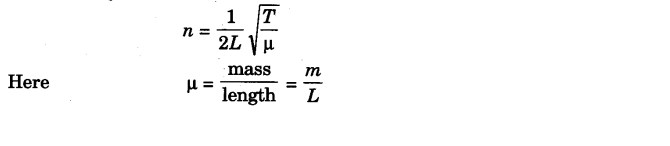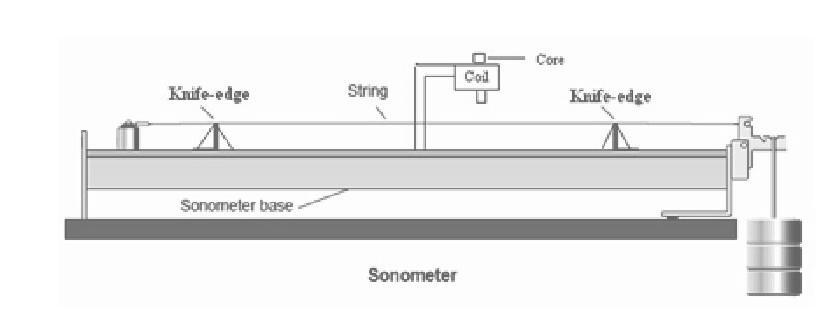# To determine frequency of A.C. Mains using Sonometer

## Objective / Aim of the Experiment

To determine frequency of A.C. Mains using Sonometer

### Apparatus Required

Sonometer (with soft iron wire)
A set of eight Tuning Forks
1/2 Kg Hanger
Seven 1/2 Kg slotted weights
Clamp stand
Paper rider
Metre scale

### Theory

Let the alternating current have frequency v so that the frequency of magnetisation of the electromagnet (VE) becomes 2v. Let a loaded stretched soft iron wire have resonant length l1 with the electromagnet. Let a tuning fork of frequency VT have resonant length l2.

Then from the law of length
VE l1 = VT l2 or VE = VT (l2 / l1)

which can be calculated, The natural frequency of oscillation for a stretched wire of length L and mass m and tension T is:### Procedure

1. Assemble the setup as shown in figure below:2. Firstly tied the wire, as its one is fixed and other end passes over pulley and carries a hanger of weights.

3. Mount the L-clamp of coil with the screws of sonometer base at a distance 2-3 mm above the wire.

4. The core of electromagnet should be lies at the center of coil.

5. Now connect mains cord between mains and sonometer.

6. Take two patch cords from the accessory box and connect the 6 V AC supply from sonometer to the coil with polarity.

7. Now hang the weight of 500gm to the hanger connected with one end of steel wire.

8. Switch on the AC supply.

9. Now adjust two knife edges near and far to each other so that you get some vibrations in wire.

10. Now slowly adjust both knife edges for maximum vibration in the wire.

11. Note the length (l) of wire between two knife edges by given meter scale.

12. Also note load (W) in kg.

13. Now increase load by 500 gm and again get the position of maximum vibration by adjusting both knife edges.

14. Again note the length (l) of wire between two knife edges.

15. Repeat the same procedure by increasing weight by 500 gm and take reading of l for maximum vibration.

16. Note all values in observation table below:

### Observation Table

Length of the wire = ................. cm = ..................... m

Mass of the wire = ................. gm = ....................kg

 S.No. Load in Kg(W) Tension in Newton T=W*9.8 Length b/w two knife edge (l) 2l (in cm) (1/2l)*100 (in 1/ meter) ( T/m) Sqrt (T/m) n = 1/2l sqrt(T/m)

Mass per meter of the wire (m) =.............................kg/m

Mean natural frequency of Sonometer Frequency n = ........... Hz

You can calculate the frequency of AC mains by putting all values in formula given below:
f = n / 2 = ....... Hz

Also calculate percentage error as standard frequency of AC mains is 50Hz.

### Sample Results

Length of the wire = 88 cm = 0.88 m

Mass of the wire = 1.8 gm= 0.0018 Kg

Mass per meter of the wire (m) =0.002 Kg

 S.No. Load in Kg(W) Tension in Newton T=W*9.8 Length b/w two knife edge (l) 2l (in cm) (1/2l)*100 (in 1/ meter) (T/m) Sqrt (T/m) n = 1/2l sqrt(T/m) 1 0.5 4.9 25.6 51.2 1.953125 2450 49.4974747 96.67476 2 1 9.8 35.6 71.8 1.39275766 4900 70 97.49304 3 1.5 14.7 39.5 79 1.265822785 7350 85.732141 108.5217 4 2 19.6 44.6 89.2 1.121076233 9800 98.9949494 110.9809

Mean natural frequency of sonometer Frequency n = 105.3337 Hz

The frequency of AC mains can be calculated by substituting the value of n in formula given below:
f = n/2 = 105.33/2 = 52.66 Hz

Percentage Error = [(Calculate value - Standard value) / Standard value] x 100%

Calculated value = 52.66 Hz

Standard frequency = 50Hz

Percentage Error = [(52.66 - 50)/50] x 100 = 5.3%

### Precautions

1) Do not use open ended wires to connect 230V, 50Hz power supply.

2) Check the connection before giving the power supply.

3) Observations should be done carefully.

4) Disconnect the circuit after switched off the power supply

### Precautions

1. All precautions of sonometer experiment should be observed.
2. The wire should be of soft iron or of any other magnetic material.
3. Tip of electromagnet should be very close to the wire in its middle*
4. Length should be noted when the amplitude of vibration is maximum.

### Sources of error

• Wire may not be rigid and of uniform cross-sectional area.
• Pulley may not be frictionless.
• Weights may not be correct.
• Knife edges (bridges) may not be sharp.
• The main frequency may not be stable.

### Sonometer Experiment Viva Questions and Answers

Question-1: What is a sonometer
Answer-1: It is an instrument which is used to study the vibrations produced by vibrating strings. It is also called monochord.

Question-2: Why is sonometer so called
Answer-1: Because it enables us to measure the frequency of vibrating body producing sound.

Question-3: Why does string of sonometer vibrate
Answer-1: When the vibrating tuning fork is kept on the board of sonometer, the forced vibrations of the board communicate energy to string which in turn is set into forced vibrations.

Question-4: Give the unit of frequency

Question-5: Give the relation between time period and frequency
Answer-1: n = 1/T where n is the frequency and T is the time period.

Question-6: Define time period
Answer-1: Time taken by a body to complete one vibration is called its time period.

Question-7: What is frequency
Answer-1: The number of vibrations produced by a vibrating body in one second is called its frequency

Question-8: Define wavelength
Answer-1: It is the distance travelled by a wave in a time during which any particle of the medium completes its one vibration.

Question-9: What is the distance between two consecutive nodes or antinodes
Answer-1: It is equal to the half of wavelength (lambda/2).

Question-10: What are antinodes
Answer-1: There are the points on the stationery waves where the displacement is maximum and strain is zero.

Question-11: What are nodes
Answer-1: Nodes are those points in the stationary waves where the particles are permanently at rest and strain is maximum.

Question-12: What are stationery waves
Answer-1: When to identical waves of same frequency and same amplitude travelling in a medium in a straight line but in opposite direction, they superimpose to each other and give rise a stationery wave.

Question-13: What types of waves are produced in the wire

Question-14: What is wave motion
Answer-1: It is a form of disturbance which travels through the medium due to the repeated periodic motion of the particles of the medium about their mean position.

Question-15: What are the types of wave motion
Answer-1: There are two types of wave motions:
1. Transverse wave motion
2. Longitudinal wave motion

Question-16: What u meant by Progressive wave
Answer-1: A disturbance created in an elastic medium propagates outwards in an elastic medium in the form of a wave, is called progressive wave.

Question-17: How many types of progressive waves are there
Answer-1: Progressive waves are of two types and they are:
(1) Transverse waves
(2) Longitudinal waves.

Question-18: Difference between transverse wave and longitudinal wave
Answer-1: In a transverse wave the particle vibrate perpendicularly where as in longitudinal wave the particle vibrate parallelly with respect to the direction of propagation of a wave.

### Latest Articles### Spreading Knowledge Across the World

USA - United States of America  Canada  United Kingdom  Australia  New Zealand  South America  Brazil  Portugal  Netherland  South Africa  Ethiopia  Zambia  Singapore  Malaysia  India  China  UAE - Saudi Arabia  Qatar  Oman  Kuwait  Bahrain  Dubai  Israil  England  Scotland  Norway  Ireland  Denmark  France  Spain  Poland  and  many more....